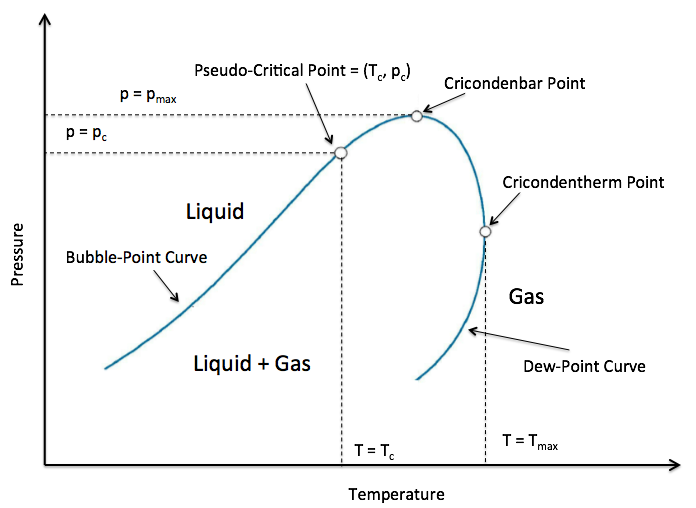##### Page tree
Go to start of banner

# Pseudo-Critical Point = (Tpc, Ppc)

A property of a Fluid Mixture represented by the
junction point of Vapour Liquid Equilibrium (VLE) area on PT diagram of Fluid Mixture (see Fig. 1).

It is characterized by pseudo-critical temperature  $//$ and pseudo-critical pressure $//$.Fig. 1. Schematic PT diagram with .

The simplest estimate  (also called Linear Blending Rule) of$//$ is the mole fraction weighted average of the critical temperature $//$ and  critical pressure $//$ of fluid components (which are pure substances) :

 (1)
 (2)

where

 $//$ mole fraction of the $//$-th mixture component $//$ critical temperature of the $//$-th mixture component $//$ critical pressure of the $//$-th mixture component

There are other no-linear methods to blend the components  to estimate the
Pseudo-Critical Point but many engineering applications use (1) and (2) as the seed and then fit the values to match lab test data.

In case the fluid composition is not known one can use empirical
Pseudo-Critical Point Correlations to assess the